Quantum encryption

NorzhCTF 2020 - Crypto (350 pts).

Quantum encryption

SUMMARY

Since Google’s announcement claiming to have achieved quantum supremacy, Norzh Nuclea has assigned one of their engineers to develop a quantum encryption algorithm.

Check the reliability of this project based on the project files you got.

TL;DR

The quantum circuit relies on X gate and swapping, but never applies quantum superposition principle.

Therefore, it allows us to implement an alternative algorithm based on classical operators such as XOR and NOT without dealing with probabilities when measuring qubits since $|\Psi\rangle = |1\rangle$ or $|\Psi\rangle = |0\rangle$.

WRITEUP

Git repo

We’ve access to a Norzh Nuclea private instance of Gogs containing a project named quantum_encryption: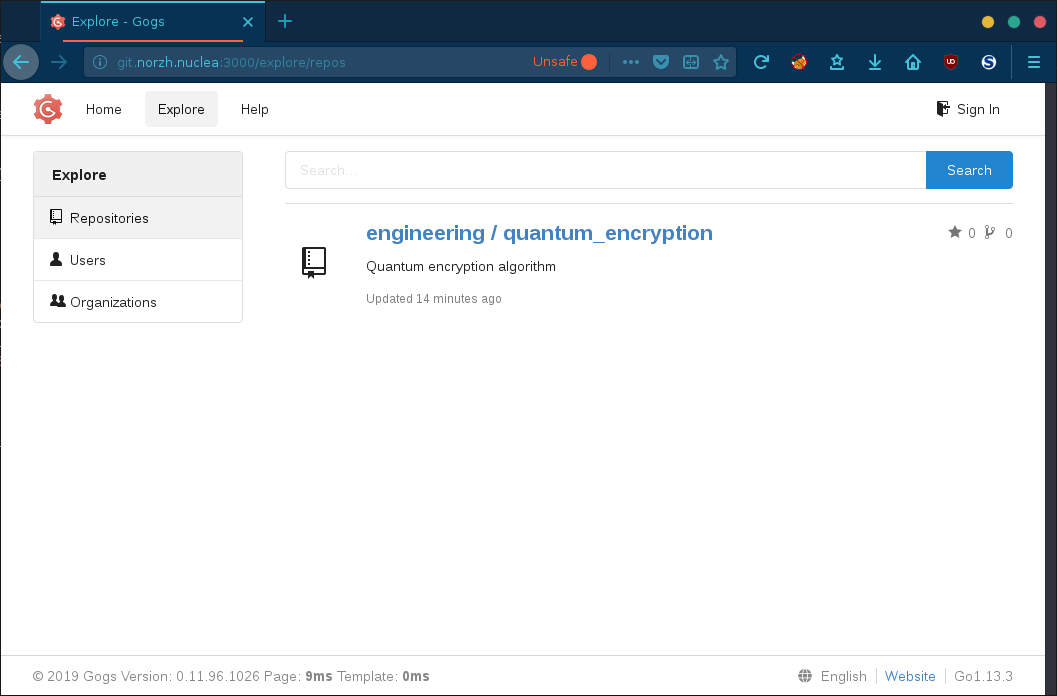Looking at the issues, we’re informed that a message has been encoded using a custom encryption algorithm: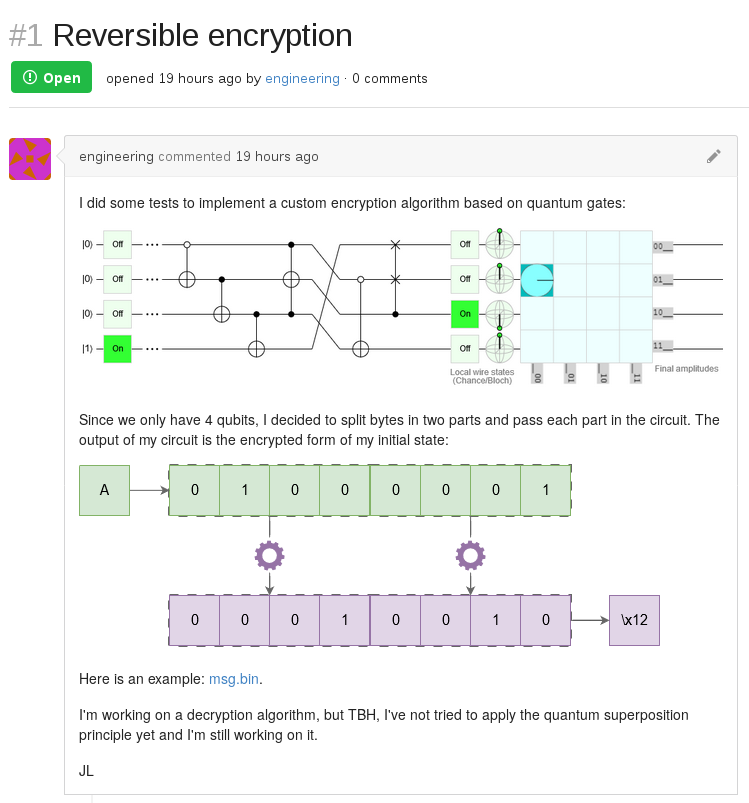Jean Luc says that he didn’t rely on the quantum superposition principle and that he encoded the message using its byte representation.

Then, each character is split into two nibbles and passed through the algorithm to get its encrypted form.

Quantum superposition principle

The quantum superposition principle states that any qubits state can be represented as a combination of distinct states.

For example, we can consider the output of the algorithm with 16 configurations:

$|0000\rangle, |0001\rangle, \cdots, |1110\rangle, |1111\rangle$

Quantum gates

Looking at the following diagram, we are not necessarily confident with all the notations and symbols used in this circuit: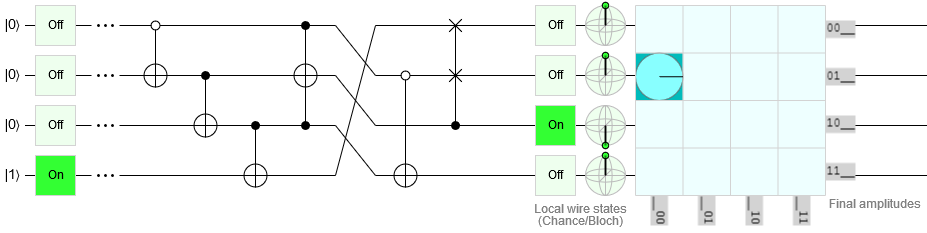Fortunately, there is a lot of documentation on the WEB (e.g. here, here and here).

Circuit’s gate decomposition

Looking at the documentation, we learn that not all quantum devices can execute all quantum gates.

In fact, some quantum gates are constructed from a series of universal quantum gates such as the Pauli-X gate, Controlled Pauli-X gate, Swap gate and Toffoli gate.

Anti-controlled X gate

The anti-controlled Pauli-X gate consists in performing a Pauli-X gate on the target qubit when the control qubit is in state $|1\rangle$.

The following truth table describes how this gate works (it’s worth noting that the truth table looks like a basic XNOR gate)

Control Input Control Output
$\vert0\rangle$ $\vert0\rangle$ $\vert0\rangle$ $\vert1\rangle$
$\vert0\rangle$ $\vert1\rangle$ $\vert0\rangle$ $\vert0\rangle$
$\vert1\rangle$ $\vert0\rangle$ $\vert1\rangle$ $\vert0\rangle$
$\vert1\rangle$ $\vert1\rangle$ $\vert1\rangle$ $\vert1\rangle$

which, when analysing the basic controlled Pauli-X gate, corresponds to the reverse state (which also looks like a basic XOR gate):

Control Input Control Output
$\vert0\rangle$ $\vert0\rangle$ $\vert0\rangle$ $\vert0\rangle$
$\vert0\rangle$ $\vert1\rangle$ $\vert0\rangle$ $\vert1\rangle$
$\vert1\rangle$ $\vert0\rangle$ $\vert1\rangle$ $\vert1\rangle$
$\vert1\rangle$ $\vert1\rangle$ $\vert1\rangle$ $\vert0\rangle$

Therefore, we can decompose this gate into the following series of universal and basic quantum gates:equals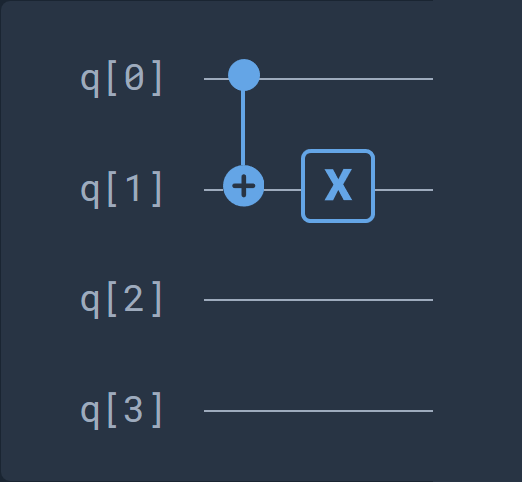Qubit left shift

Looking at the following circuit part: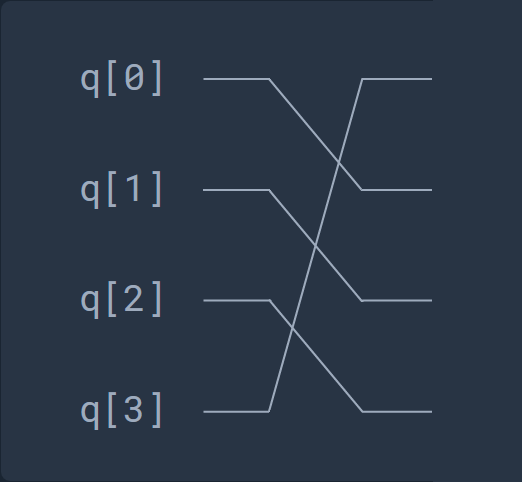we can see that it consists in basic series of swap gates since the qubits values are swapped between multiple qubits. Here is an equivalent circuit: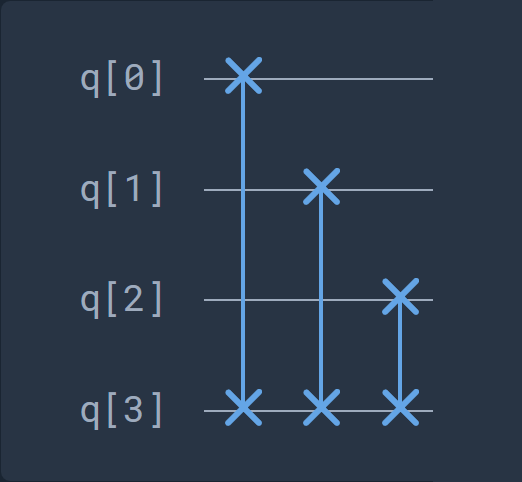Fredkin gate

The last device-specific gate is the following one: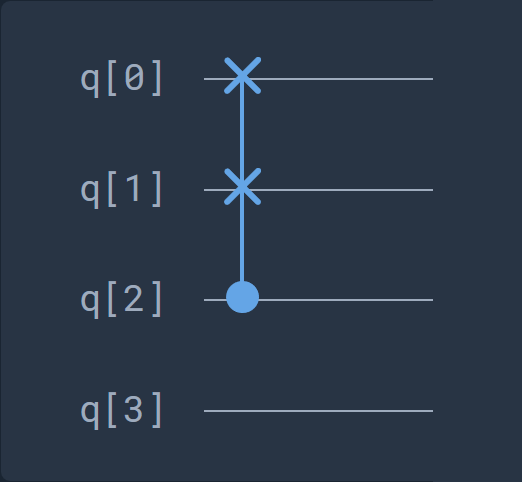This gate is a Fredkin gate, which is a controlled swap gate that maps three inputs onto three outputs:

Control Input 1 Input 2 Control Output 1 Output 2
$\vert0\rangle$ $\vert0\rangle$ $\vert0\rangle$ $\vert0\rangle$ $\vert0\rangle$ $\vert0\rangle$
$\vert0\rangle$ $\vert0\rangle$ $\vert1\rangle$ $\vert0\rangle$ $\vert0\rangle$ $\vert1\rangle$
$\vert0\rangle$ $\vert1\rangle$ $\vert0\rangle$ $\vert0\rangle$ $\vert1\rangle$ $\vert0\rangle$
$\vert0\rangle$ $\vert1\rangle$ $\vert1\rangle$ $\vert0\rangle$ $\vert1\rangle$ $\vert1\rangle$
$\vert1\rangle$ $\vert0\rangle$ $\vert0\rangle$ $\vert1\rangle$ $\vert0\rangle$ $\vert0\rangle$
$\vert1\rangle$ $\vert0\rangle$ $\vert1\rangle$ $\vert1\rangle$ $\vert1\rangle$ $\vert0\rangle$
$\vert1\rangle$ $\vert1\rangle$ $\vert0\rangle$ $\vert1\rangle$ $\vert0\rangle$ $\vert1\rangle$
$\vert1\rangle$ $\vert1\rangle$ $\vert1\rangle$ $\vert1\rangle$ $\vert1\rangle$ $\vert1\rangle$

Here is an equivalent series for this gate: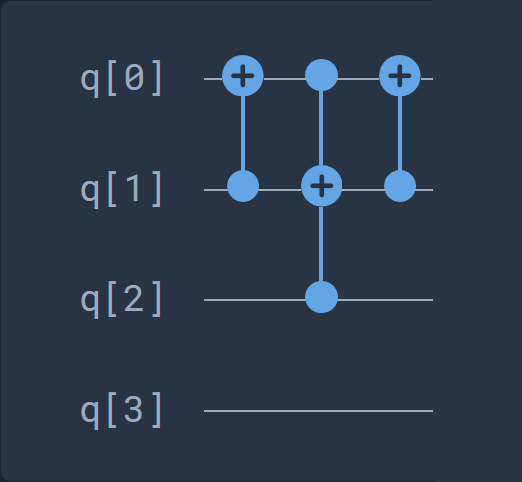Decomposed circuit

The following diagram represents a quantum circuit equivalent to the initial circuit: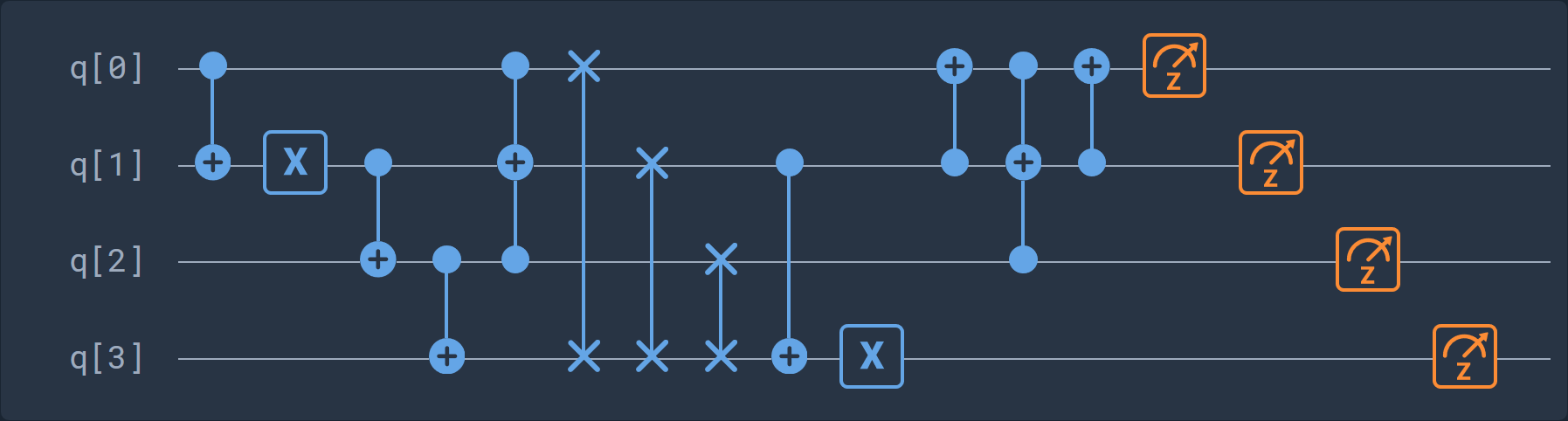Here is the corresponding cQASM 1.0 code:

version 1.0

qubits 4

CNOT q,q # CNOT gate between qubits 0 and 1
X q # execute x-gate on qubit 1
CNOT q,q # CNOT gate between qubits 1 and 2
CNOT q,q # CNOT gate between qubits 2 and 3
Toffoli q,q,q # Toffoli gate between qubits 0,2 and 1
SWAP q,q # SWAP gate on qubits 0 and 3
SWAP q,q # SWAP gate on qubits 1 and 3
SWAP q,q # SWAP gate on qubits 2 and 3
CNOT q,q # CNOT gate between qubits 1 and 3
X q # execute x-gate on qubit 3
CNOT q,q # CNOT gate between qubits 1 and 0
Toffoli q,q,q # Toffoli gate between qubits 0,2 and 1
CNOT q,q # CNOT gate between qubits 1 and 0
measure q # Measurement on qubit 0 in the z-basis
measure q # Measurement on qubit 1 in the z-basis
measure q # Measurement on qubit 2 in the z-basis
measure q # Measurement on qubit 3 in the z-basis

It’s worth noting that we had access to the equivalent OpenQASM code which is a little bit different:

// quantum encryption
OPENQASM 2.0;
include "qelib1.inc";

qreg q;
creg c;

cx q,q;
x q;
cx q,q;
cx q,q;
ccx q,q,q;
swap q,q;
swap q,q;
swap q,q;
cx q,q;
x q;
cx q,q;
ccx q,q,q;
cx q,q;
measure q -> c;
measure q -> c;
measure q -> c;
measure q -> c;

According to documentation about the logic gates reversibility, we can confirm that this algorithm is reversible and that we should be able to get the clear text from its encrypted form.

Decryption circuit

We now know how our message is encrypted (in fact it’s encoded here), that the circuit is reversible, that the superposition principle is not applied and that we only work with classical bits. Now, let’s put all the pieces together and create a decryption circuit: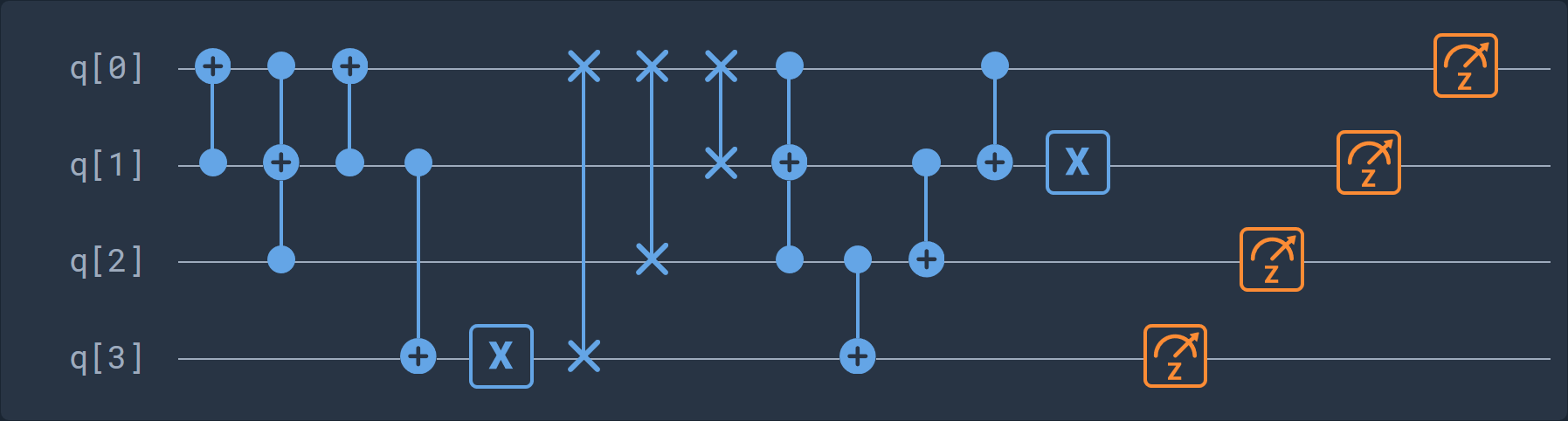Its corresponding cQASM code:

version 1.0

qubits 4

CNOT q,q # CNOT gate between qubits 1 and 0
Toffoli q,q,q # Toffoli gate between qubits 0,2 and 1
CNOT q,q # CNOT gate between qubits 1 and 0
CNOT q,q # CNOT gate between qubits 1 and 3
X q # execute x-gate on qubit 3
SWAP q,q # SWAP gate on qubits 3 and 0
SWAP q,q # SWAP gate on qubits 2 and 0
SWAP q,q # SWAP gate on qubits 1 and 0
Toffoli q,q,q # Toffoli gate between qubits 0,2 and 1
CNOT q,q # CNOT gate between qubits 2 and 3
CNOT q,q # CNOT gate between qubits 1 and 2
CNOT q,q # CNOT gate between qubits 0 and 1
X q # execute x-gate on qubit 1
measure q # Measurement on qubit 3 in the z-basis
measure q # Measurement on qubit 2 in the z-basis
measure q # Measurement on qubit 1 in the z-basis
measure q # Measurement on qubit 0 in the z-basis

And its corresponding OpenQASM code:

OPENQASM 2.0;
include "qelib1.inc";

qreg q;
creg c;

cx q,q;
ccx q,q,q;
cx q,q;
cx q,q;
x q;
swap q,q;
swap q,q;
swap q,q;
ccx q,q,q;
cx q,q;
cx q,q;
cx q,q;
x q;
measure q -> c;
measure q -> c;
measure q -> c;
measure q -> c;

Scripting

In order to get a quick result for decrypting our message, we can create a script:

#!/usr/bin/env python3
# -*- coding: latin-1 -*-
from collections import deque

def bin_str_to_list(state):
'''
Get a bit list from a binary string.
'''
return list(map(lambda x: int(x), list(state)))

def bin_list_to_str(state):
'''
Get a binary string from a bit list.
'''
return ''.join(map(lambda x: str(int(x)), state)).zfill(4)

def byte_to_nibbles(byte):
'''
Split the byte into high and low nibbles.
'''
return (byte >> 4, byte & 0x0F)

def CX(t, c):
'''
Pauli gate / Controlled-X gate.
'''
t ^= c
return (t, c)

def reverse_CX(t, c):
'''
Reverse Pauli gate / Reverse controlled-X gate.
'''
return (CX(t, not c), c)

def CCX(t, c1, c2):
'''
Toffoli gate / Controlled-Controlled-X gate.
'''
return (CX(t, (c1 & c2)), c1, c2)

def CSWAP(t1, t2, c):
'''
Fredkin gate / Controlled-SWAP.
'''
t1, t2 = CX(t1, t2)
t2, t1, c = CCX(t2, t1, c)
t1, t2 = CX(t1, t2)
return (t1, t2, c)

def decode(state):
if len(state) == 4:
state = deque(bin_str_to_list(state))

# - CSWAP - #
state, state, state = CSWAP(state, state, state)

# - reverse CNOT / reverse CX - #
state, state = reverse_CX(state, state)

# SWAP(t4, t1); SWAP(t3, t1); SWAP(t2, t1) = right rotate
state.rotate(-1)

# - CCNOT / CCX - #
state, state, state = CCX(state, state, state)

# - CNOT / CX - #
state, state = CX(state, state)

# - CNOT / CX - #
state, state = CX(state, state)

# - reverse CNOT / reverse CX - #
state, state = reverse_CX(state, state)

return state

def main():
with open('msg.bin', 'rt', encoding='latin-1') as fd:
buffer = list()
for i in range(len(flag_enc)):  # for each bytes
buffer.append('')  # add an entry to the buffer
byte = ord(flag_enc[i])  # get byte
nibbles = byte_to_nibbles(byte)  # split the byte into high low nibbles
for j in range(2):  # for each nibbles
state = bin(nibbles[j])[2:].zfill(4)  # convert the nibble to a binary string
out_state = decode(state)  # get the output state of decoder
buffer[i] += bin_list_to_str(out_state)  # add the nibble to buffer
flag = list(map(lambda b: chr(int(b, 2)), buffer))  # for each bytes in buffer, get its char value
print(''.join(flag))

if __name__ == "__main__":
main()

FLAG

Final flag is: ENSIBS{qu4ntUm_G4tEs_w1ThOU7_SuPErp0si71oN}

Happy Hacking!

Creased